# JEE Main Capacitor Previous Year Questions with Solutions

Capacitor is a device which is used to store energy. The capacitor is an arrangement in which an insulator separates two conductors kept near each other. The insulating medium of the capacitor can be air, paper, glass or a dielectric material. Capacitors come in different shapes and sizes. The amount of electric charge that can be stored in the capacitor per unit voltage across its plates is called capacitance.

Download Capacitor Previous Year Solved Questions PDF

## Application of Capacitors

Capacitors have a wide range of applications. Some applications are given below:

• Capacitors are used in computers to store a large amount of data.
• Sensitive components of electric surges are protected by using capacitors as filters.
• Air humidity, fuel levels, etc can be measured by using capacitors as sensors.
• Capacitor coupling is the process of effectively blocking DC current and passing AC current using capacitors.
• Unwanted signals are filtered using capacitors.

## JEE Main Previous Year Solved Questions on Capacitor

Q1: A parallel plate capacitor with plates of area 1 m2 each are at a separation of 0.1 m. If the electric field between the plates is 100 N C–1, the magnitude of charge on each plate is

(Taken ε0 = 8.85 x 10-12 C2/N m2)

(a) 8.85 × 10–10 C

(b) 7.85 × 10–10 C

(c) 9.85 × 10–10 C

(d) 6.85 × 10–10 C

Solution

The electric field between two plates is

E = σ/ε0 = q/Aε0 ⇒q = EAε0

q = (100)(1)(8.85 x 10-12)= 8.85 × 10–10 C

Answer: (a) 8.85 × 10–10 C

Q2: The parallel combination of two air filled parallel plate capacitors of capacitance C and nC is connected to a battery of voltage, V. When the capacitors are fully charged, the battery is removed and after that a dielectric material of dielectric constant K is placed between the two plates of the first capacitor. The new potential difference of the combined system is

(a) V

(b) (n+1)V/(K+n)

(c) (nV)/(K+n)

(d) V/(K+n)

Solution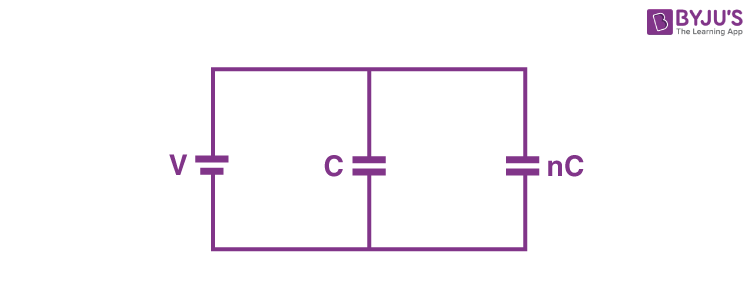For parallel combination, Ceq = C + nC = C(n + 1)

Charge on capacitor, q = CeqV = CV(n + 1)

Now, after removing the battery, dielectric material is placed.

Then, Ceq = KC + nC = C(K + n)

New potential difference, V’ = q/Ceq =CV(n+1)/C(K+n) = (n+1)V/(K+n)

Q3: A parallel plate capacitor is made by stacking n equally spaced plates connected alternatively. If the capacitance between any two adjacent plates is C then the resultant capacitance is

(a) C

(b) nC

(c) (n – 1)C

(d) (n + 1)C

Solution

n plates connected alternately give rise to (n – 1) capacitors connected in parallel

Resultant capacitance = (n – 1)C.

Answer: (c) (n – 1)C

Q4: Capacitance (in F) of a spherical conductor with radius 1 m is

(a) 1.1 × 10–10

(b) 10–6

(c) 9 × 10–9

(d) 10–3

Solution

C = 4πε0R = 1/9 x 109 = 1.1 x 10-10 F

Answer: (a) 1.1 × 10–10

Q5: If there are n capacitors in parallel connected to V volt source, then the energy stored is equal to

(a) CV

(b) (½)nCV2

(c) CV2

(d) (1/2n)CV2

Solution

Total capacity =nC

∴ Energy =½ nCV2

Q6: A parallel plate capacitor with air between the plates has a capacitance of 9 pF. The separation between its plates is d. The space between the plates is now filled with two dielectrics. One of the dielectrics has dielectric constant k1 = 3 and thickness d/3 while the other one has dielectric constant k2 = 6 and thickness 2d/3. The capacitance of the capacitor is now

(a) 20.25 pF

(b) 1.8 pF

(c) 45 pF

(d) 40.5 pF

Solution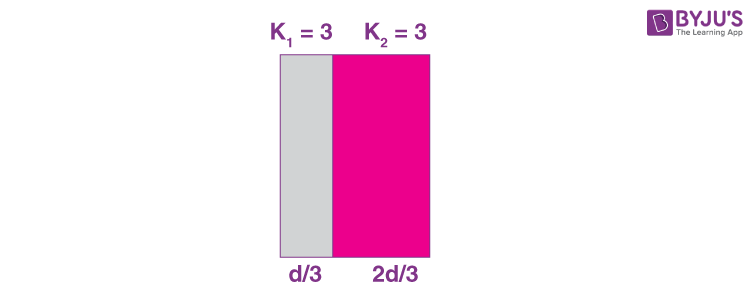C = ε0A/d = 9 x 10-12 F

With dielectric,C = ε0Ak/d

C1 = ε0A3/(d/3) = 9C

C2 = ε0A6/(2d/3) = 9C

Ctotal = C1C2/(C1 + C2) as they are in series

Ctotal = (9C x 9C)/18C = (9/2)C =(9/2)(9 x 10-12 F)

Ctotal = 40.5 pF

Answer: (d) 40.5 pF

Q7: A parallel plate condenser with a dielectric of dielectric constant K between the plates has a capacity C and is charged to a potential V volt. The dielectric slab is slowly removed from between the plates and then reinserted. The net work done by the system in this process is

(a) zero

(b) 1/2 (K – 1) CV2

(c) CV2(K-1)/K

(d) (K – 1) CV2

Solution

The potential energy of a charged capacitor

Ui = q2/2C

where Ui is the initial potential energy.

If a dielectric slab is slowly introduced, the energy = q2/2KC

Once it is taken out, again the energy increases to the old value. Therefore after it is taken out, the potential energy comes back to the old value.

Total work done = zero.

Q8: A battery is used to charge a parallel plate capacitor till the potential difference between the plates becomes equal to the electromotive force of the battery. The ratio of the energy stored in the capacitor and the work done by the battery will be

(a) 1/2

(b) 1

(c) 2

(d) 1/4

Solution

Let E be emf of the battery

Work done by the battery W = CE2

Energy stored in the capacitor

U = ½CE2

U/W = ½CE2 /CE2

Q9: A sheet of aluminium foil of negligible thickness is introduced between the plates of a capacitor. The capacitance of the capacitor

(a) decreases

(b) remains unchanged

(c) becomes infinite

(d) increases

Solution

With air as dielectric, C = ε0A/d

With space partially filled, C’ = ε0A/(d-t) = ε0A/d = C

Aluminium is a good conductor. Its sheet introduced between the plates of a capacitor is of negligible thickness. Therefore,  capacity remains unchanged.

Answer: (b) remains unchanged

Q10: If the electric flux entering and leaving an enclosed surface respectively is Φ1 and Φ2, the electric charge inside the surface will be

(a) (Φ2Φ1)ε0

(b) (Φ1 + Φ2)/ε0

(c) (Φ2Φ1)/ε0

(d) (Φ1 + Φ2)ε0

Solution

According to Gauss theorem,

2 – Φ1) = Q/ε0

⇒ Q = (Φ2 – Φ10

The flux enters the enclosure if one has a negative charge (–q2) and flux goes out if one has a +ve charge (+q1). As one does not know whether Φ1 > Φ2, Φ2 > Φ1, Q = q1 ~ q2

Q11: A parallel plate capacitor is of area 6 cm2 and a separation of 3 mm. The gap is filled with three dielectric materials of equal thickness (see figure) with dielectric constants K1 = 10, K2 = 12 and K3 = 14. The dielectric constant of a material which when fully inserted in the given capacitor gives the same capacitance would be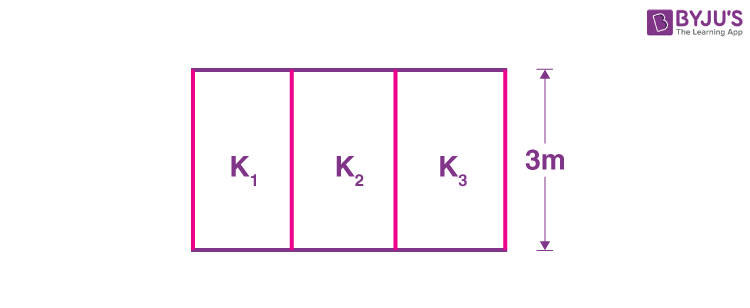(a) 36

(b) 12

(c) 4

(d) 14

Solution

The given system can be considered to be a parallel combination of three capacitors C1, C2 and C3. The equivalent capacitance, C = C1 + C2 + C3

Hence, C1 = K1ε0A/3d

C2 = K2ε0A/3d

C3 = K3ε0A/3d

(K’ε0A/d) = (K1 + K2 + K3/3)(ε0A/d)

Hence K’= (K1 + K2 + K3/3) = (10 + 12 + 14/3) =12

Q12: In the figure shown below, the charge on the left plate of the 10 F capacitor is 30 μC. The charge on the right plate of the 6 F capacitor is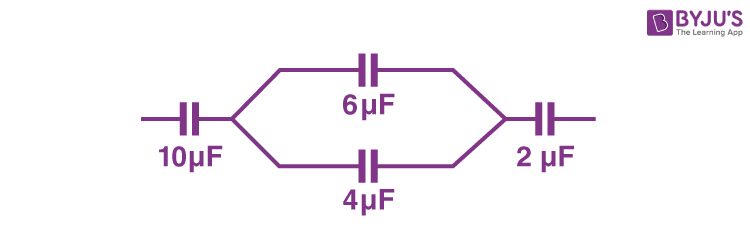(a) –18 μC

(b) –12 μC

(c) +12 μC

(d) +18 μC

Solution

Let q1 and q2 be the charge on 6μF and 4μF respectively

q1 + q2 = q——-(1)

Also, (q1/C1) = (q2/C2)——-(2)

(∵ C1 and C2 are in parallel combination)

⇒ q2 = (C2/C1) q1= (4/6)q1

Using (1) and (2)

(10/6)q1 = q ⇒ q = (5/3)

⇒q1=  (⅗)(30) = 18 μC

Answer: (d) +18 μC

Q13: Seven capacitors, each of capacitance 2 F, are to be connected in a configuration to obtain an effective capacitance of (6/13) F. Which of the combinations, shown in figures below, will achieve the desired value?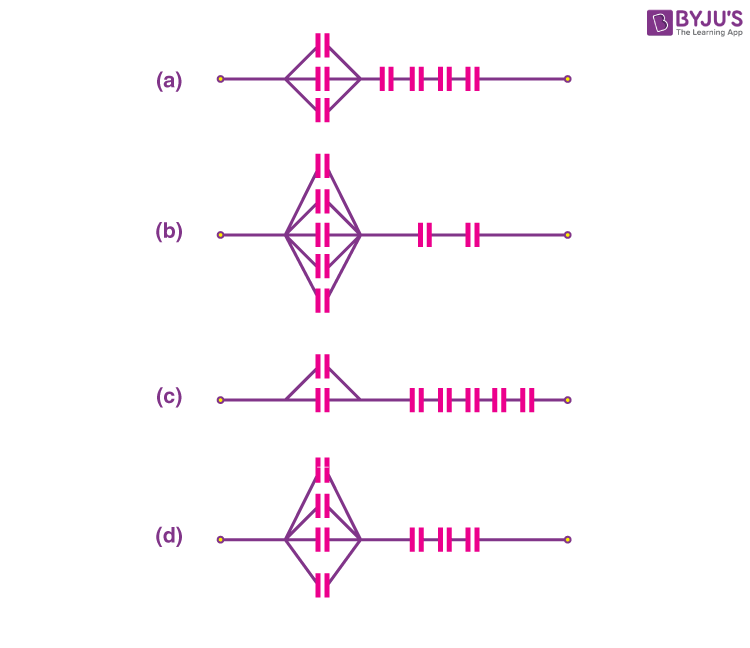Solution

Equivalent capacitance for parallel capacitors is given by the formula

Ceq= C1 + C2 +C3 ….

Therefore, 1/Ceq = 1/(C1 + C2 + C3)

Equivalent capacitance for series capacitors is given by the formula

1/Ceq = 1/C1 + 1/C2 +1/C3

In option “a” there are 3 parallel capacitors and 4 series capacitors

Therefore, Equivalent capacitance = 1/(2+2 +2) + (1/2) + (1/2) +(1/2) +(1/2)

= 1/6 + (1/2) + (1/2) +(1/2) +(1/2)

= 1+3+3+3+3/6

1/Ceq= 13/6 F

Ceq = (6/13) F

In option “b” there are 5 parallel capacitors and 2 series capacitors

Therefore, Equivalent capacitance = 1/(2+2 +2+2+2) + (1/2) + (1/2)

= 1/10+ (1/2) + (1/2)

= 1+5+5/10

1/Ceq= 11/10 F

Ceq = 10/11 F

In option “c” there are 2 parallel capacitors and 5 series capacitors

Therefore, Equivalent capacitance = 1/(2+2)  + (1/2) + (1/2)+ (1/2) + (1/2) +(1/2)

= 1/4+ (1/2) + (1/2)+ (1/2) + (1/2) +(1/2)

= 1+2+2+2+2+2/4

1/Ceq= 11/4 F

Ceq = (4/11) F

In option “d” there are 4 parallel capacitors and 3 series capacitors

Therefore, Equivalent capacitance = 1/(2+2+2+2)  + (1/2) + (1/2)+ (1/2)

= 1/8+ (1/2) + (1/2)+ (1/2)

= 1+4+4+4/4

1/Ceq = 13/8 F

Ceq = (8/13) FQ14: Voltage rating of a parallel plate capacitor is 500 V. Its dielectric can withstand a maximum electric field of 106 V m–1. The plate area is 10–4 m2. What is the dielectric constant if the capacitance is 15 pF? (given ε0 = 8.86 × 10–12 C2 N–1 m–2)

(a) 3.8

(b) 8.5

(c) 6.2

(d) 4.5

Solution

C = Kε0A/d or K = CV/ε0AEmax

K = (15 x 10-12 x 500)/(8.86 x 10-12 x 10-4 x 106) = 8.5

Q15:In free space, a particle A of charge 1 μC is held fixed at a point P. Another particle B of the same charge and mass 4 g is kept at a distance of 1 mm from P. If B is released, then its velocity at a distance of 9 mm from P is

(take 1/4πε0 = 9 x 109 Nm2C-2)

(a) 3.0 × 104 m/s

(b) 1.0 m/s

(c) 1.5 × 102 m/s

(d) 2.0 × 103 m/s

Solution

Using work energy theorem

WE = Ui – Uf= (½)mv2 or kq1q2[(1/r1) – (1/r2)] = (½)mv2

WE = (9 x 109) x (1 x 10-6)2 {(1/10-3) – (1/(9 x 10-3)} = (½)mv2

⇒ (9 x 109 x 2 x 10-12)/(4 x 10-6) = v2 ⇒ v = 2.0 x 103 ms-1

Answer: (d) 2.0 × 103 m/s

Current Electricity JEE Advanced Previous Year Questions With Solutions

## Introduction to Capacitors and Combination of Capacitors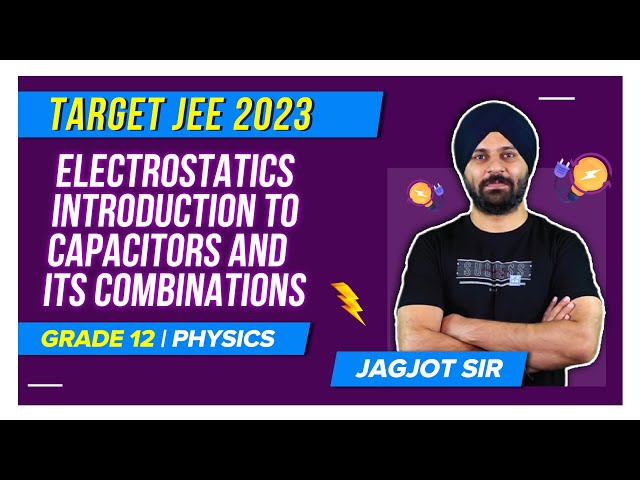## Capacitors – Video Lesson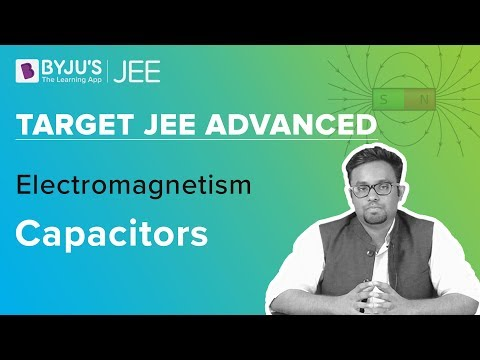## Top 10 Important Questions of Electrostatic Potential and Capacitance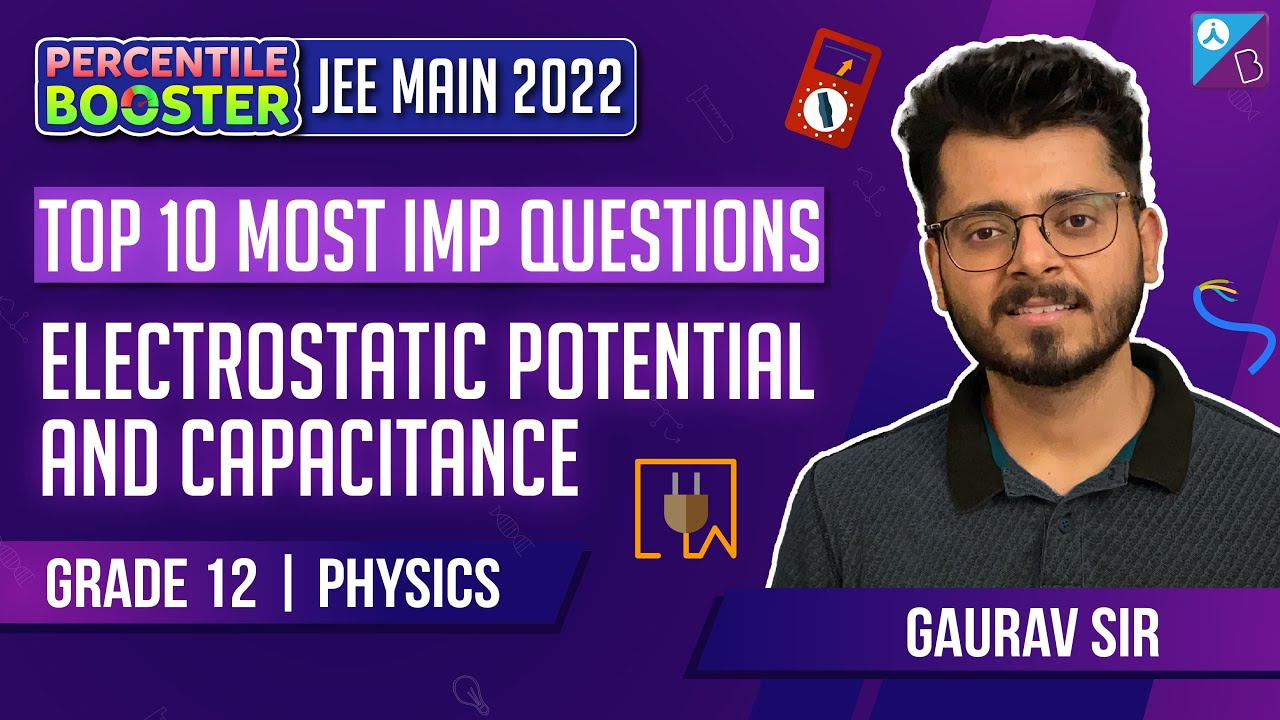Test your Knowledge on Capacitor